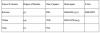# Frequently Asked Interview Questions for Black Belts_ANOVA (Basics)_SOLVED

## Recommended Posts

Dear All,

These are few frequently asked Black Belt interview questions on ANOVA Basics. Let's get answers to these questions.

1.ANOVA Table

• Why is ANOVA called Analysis of Variances when it compares means of more than two groups?
• What does the Sum of Squares (SS) represent?
• What is MS and what does it represent?
• How do we get F and what does it mean?
• How do we get the p-value?

2.Comparisons or Post Hocs

• Why do we use multiple comparisons?
• What is the difference between Tukey and Hsu's MCB?
• What is the difference Tukey and Fisher comparisons?

Everybody is invited to post answers to these questions.

Regards,

shantanu kumar

##### Share on other sites

Hi

Can u help me out with the green belt interview questions.

Thanks & Regards

Ria

##### Share on other sites

Hi Ria,

I am currently compiling frequently asked Green Belt interview questions. I will try to publish it by December'09.

Regards,

shantanu kumar

##### Share on other sites
• 3 weeks later...

Why is ANOVA called Analysis of Variances when it compares means of more than two samples?

Answer: To determine whether means of several groups are equal or not or whether the samples belong to the same population or not, ANOVA compares

• the variance of the group means(between groups), with
• the variance of values within the groups (within group)

Mathematically in a balanced ANOVA design,

a. Calculate the variance of each group and then calculate the mean of the variances - this will give you the ‘error variance' ---------(MSW)

b. Calculate the arithmetic mean of each group and then calculate the variance of the arithmetic means of the groups - this will give you ‘variance of sample means'

c. Multiply the ‘variance of sample mean' by sample size of one group-----(MSB)

d. F= (sample size*variance of sample mean)/ error variance OR MSB/MSW

ANOVA TableWhat does the Sum of Squares (SS) represent?

The sum of squares between groups (BSS) measures the variation between the group means

The sum of squares within groups (WSS) measures the variation of values within each group

What is MS and what does it represent?

Mean Squares Between = Sum of Squares between / (no of groups-1), Mean Squares Within= Sum of Squares within / (total observations - no of groups).

How do we get F and what does it mean?

It is simply the ratio of two variance estimates. F = (sample size*variance of sample mean)/ error variance OR Mean Squares between groups/ Mean Squares with group.

How do we get the p-value?

p value in ANOVA is F distribution's probability density function of x= F statistic with numerator df between group and denominator df within group. To find the p-value, you can use MS-Excel by inserting the function ‘fdist (F, df between groups, df within group)'. In Minitab, follow this path - Calc>>Probability distribution>>F distribution.

Comparisons or Post Hocs

Why do we use multiple comparisons?

ANOVA only indicates whether there are differences between one or more pair of treatment means. It doesn't indicate which pairs are different. Multiple Comparisons are used to identify significant differences between specific factor levels

What is the difference between Tukey and Hsu's MCB?

Tukey's method or the Tukey-Kramer method is used to compare all possible pairs of treatments while controlling the family error rate. HSU's method compares the best treatment (the one with the lowest or highest mean) to all other treatments while controlling the family error rate.

What is the difference Tukey and Fisher comparisons?

Fisher's method is used to compare all possible pairs of treatments using a specified error rate for individual tests. It doesn't control the family error rate. Therefore it is advised that we use Fisher's method with appropriate Bonferroni corrected alpha if only a subset of all possible comparisons is of interest.

## Join the conversation

You can post now and register later. If you have an account, sign in now to post with your account.×   Pasted as rich text.   Paste as plain text instead

Only 75 emoji are allowed.

×   Your previous content has been restored.   Clear editor

×   You cannot paste images directly. Upload or insert images from URL.

×

• ### Forum Statistics

• Total Topics
2,867
• Total Posts
14,508
• ### Member Statistics

• Total Members
55,051
• Most Online
888